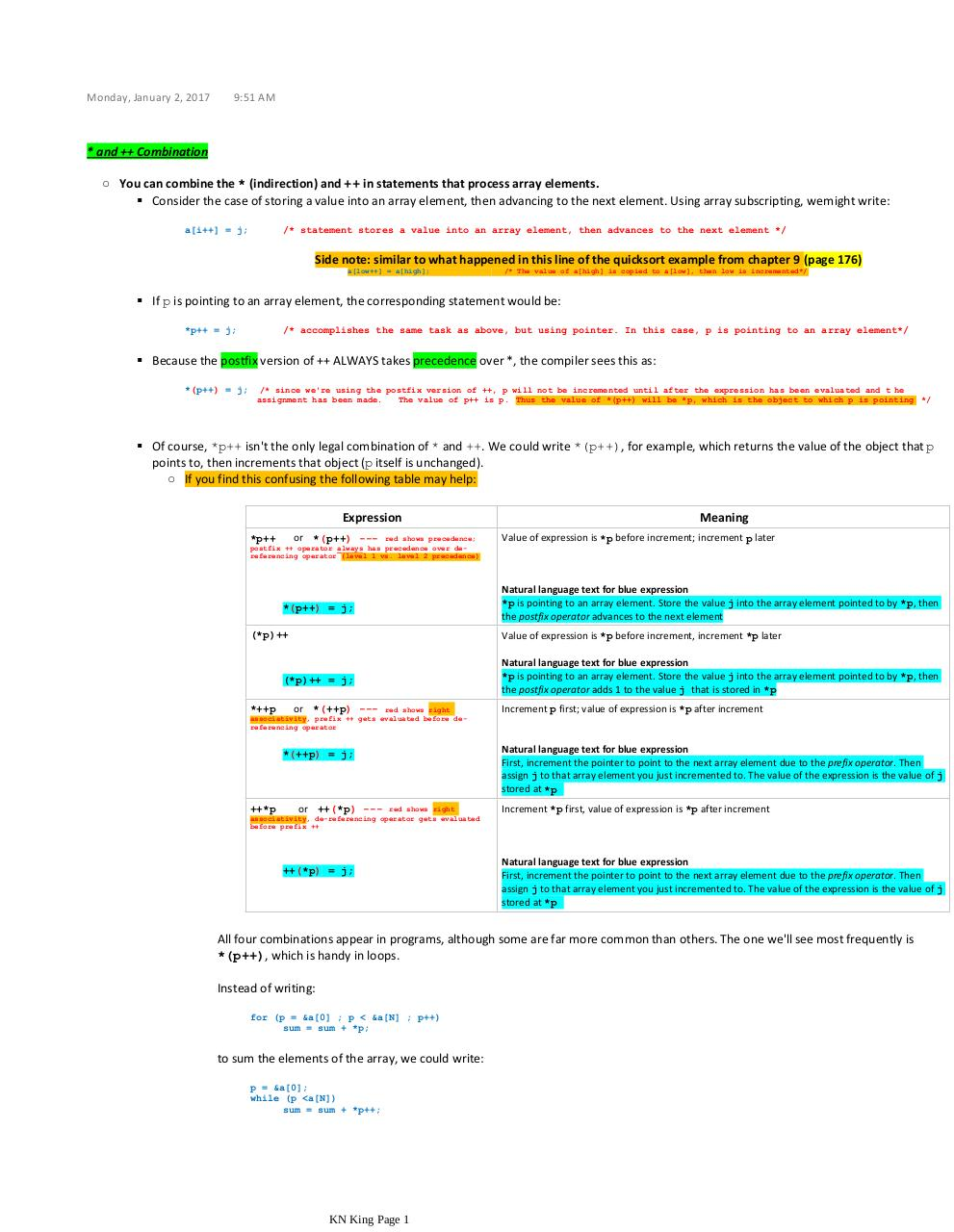# and ++ Combination .pdf

### File information

Original filename: and ++ Combination.pdf

This PDF 1.5 document has been generated by MicrosoftÂ® OneNoteÂ® 2016, and has been sent on pdf-archive.com on 02/01/2017 at 15:58, from IP address 96.255.x.x. The current document download page has been viewed 381 times.
File size: 668 KB (1 page).
Privacy: public file

and ++ Combination.pdf (PDF, 668 KB)

### Document preview

Monday, January 2, 2017

9:51 AM

* and ++ Combination
○ You can combine the * (indirection) and ++ in statements that process array elements.
 Consider the case of storing a value into an array element, then advancing to the next element. Using array subscripting, we might write:
a[i++] = j;

/* statement stores a value into an array element, then advances to the next element */

Side note: similar to what happened in this line of the quicksort example from chapter 9 (page 176)
a[low++] = a[high];

/* The value of a[high] is copied to a[low], then low is incremented*/

 If p is pointing to an array element, the corresponding statement would be:
*p++ = j;

/* accomplishes the same task as above, but using pointer. In this case, p is pointing to an array element*/

 Because the postfix version of ++ ALWAYS takes precedence over *, the compiler sees this as:
*(p++) = j;

/* since we're using the postfix version of ++, p will not be incremented until after the expression has been evaluated and t he
The value of p++ is p. Thus the value of *(p++) will be *p, which is the object to which p is pointing */

 Of course, *p++ isn't the only legal combination of * and ++. We could write *(p++), for example, which returns the value of the object that p
points to, then increments that object (p itself is unchanged).
○ If you find this confusing the following table may help:
Expression
or *(p++) ---

red shows precedence;
*p++
postfix ++ operator always has precedence over dereferencing operator (level 1 vs. level 2 precedence)

*(p++) = j;
(*p)++

Meaning
Value of expression is *p before increment; increment p later

Natural language text for blue expression
*p is pointing to an array element. Store the value j into the array element pointed to by *p, then
the postfix operator advances to the next element
Value of expression is *p before increment, increment *p later

(*p)++ = j;
*++p

or *(++p) --- red shows right
associativity, prefix ++ gets evaluated before dereferencing operator
*(++p) = j;

++*p

or ++(*p) --- red shows right
associativity, de-referencing operator gets evaluated
before prefix ++

++(*p) = j;

Natural language text for blue expression
*p is pointing to an array element. Store the value j into the array element pointed to by *p, then
the postfix operator adds 1 to the value j that is stored in *p
Increment p first; value of expression is *p after increment

Natural language text for blue expression
First, increment the pointer to point to the next array element due to the prefix operator. Then
assign j to that array element you just incremented to. The value of the expression is the value of j
stored at *p
Increment *p first, value of expression is *p after increment

Natural language text for blue expression
First, increment the pointer to point to the next array element due to the prefix operator. Then
assign j to that array element you just incremented to. The value of the expression is the value of j
stored at *p

All four combinations appear in programs, although some are far more common than others. The one we'll see most frequently is
*(p++), which is handy in loops.

for (p = &amp;a ; p &lt; &amp;a[N] ; p++)
sum = sum + *p;

to sum the elements of the array, we could write:
p = &amp;a;
while (p &lt;a[N])
sum = sum + *p++;

KN King Page 1